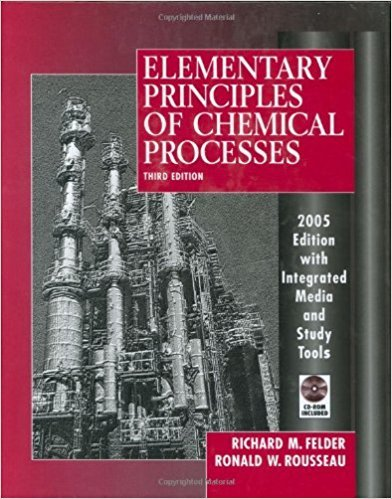×
Get Full Access to Elementary Principles Of Chemical Processes - 3 Edition - Chapter 9 - Problem 9.5
Get Full Access to Elementary Principles Of Chemical Processes - 3 Edition - Chapter 9 - Problem 9.5

×

# Use Hess's law to calculate the standard heat of theISBN: 9780471687573 143

## Solution for problem 9.5 Chapter 9

Elementary Principles of Chemical Processes | 3rd Edition

• Textbook Solutions
• 2901 Step-by-step solutions solved by professors and subject experts
• Get 24/7 help from StudySoup virtual teaching assistantsElementary Principles of Chemical Processes | 3rd Edition

4 5 1 363 Reviews
16
0
Problem 9.5

Use Hess's law to calculate the standard heat of the water-gas shift reaction CO(g) + HzO(v) -+ COz(g) + H2(g) from each of the two sets of data given here. (a) CO(g) + HzO(I) --. COz(g) + Hz(g): !:lil~ = +1226 Btu/lb-mole HzO(1) --. HzO(v): !:lHv = +18,935 Btu/lb-mole (b) CO(g) + Oz(g) --. COz(g): !:lH~ = -121,740 Btu/lb-mole Hz(g) + i Oz(g) --. HzO(v): !:lH~ = -104,040 Btu/lb-mole

Step-by-Step Solution:
Step 1 of 3

Chapter 4 VII. drawing Lewis dot structures for polyatomic ion A. the charge of the ion should be taken into account when placing the valence electrons B. Sulfate will have two extra valence electrons because it has a -2 charge VIII. resonance- when two or more electron dot structures can be written with multiple bonds A. hybrid= mix of two structures IX. properties of ionic bonds: solid at room temperature, high melting and billing point, good conductors, no prefixes, are metals and non-metals, transfer electrons Properties of covalent bonds: solids, gases or liquids at room temperature, low melting and boiling point, non-conductors, uses prefixes, 2 non-metals, shared electrons

Step 2 of 3

Step 3 of 3

##### ISBN: 9780471687573

Unlock Textbook Solution